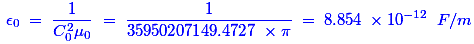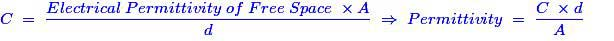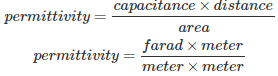# MCQs on Engineering Materials

##### Page 5 of 8. Go to page 1 2 3 4 5 6 7 8
01․ The dielectric strength of ferroelectric materials depends to a large extent on
area of hysteresis loop for the material.
presence of magnetic materials in the vicinity.
intensity of electric field.
frequency of applied voltage.

The dielectric strength of ferroelectric materials depends to a large extent on intensity of electric field applied.

02․ The absolute permittivity of free space is given by
8.854 × 10-12 F / m.
8.854 × 10-10 F / m.
8.854 × 10-11 F / m.
8.854 × 10-9 F / m.

It is an electrical constant or ideal physical constant, which is the value of the absolute dielectric permittivity of classical vacuum. The expression of the absolute permittivity is as follows,Here, C0 is the speed of light in free space, μ0 is the vacuum permeability.

03․ Permittivity is expressed in
Weber / m.
Weber / sq-m.

The electrical capacitance that is measured in farads is the only electrical property of the free space between two parallel plates of cross-section A in m2 separated by distance d in m. Thus, the expression of capacitor is as follows;Hence, the unit is Farad per meter.

04․ The potential gradient across the material of low permittivity is ______________ than across the material of high permittivity.
greater.
smaller.
both (A) and (B).
none of above.

We know that the force development by a charged particle is F = Q / (4πεd2).The electric force F and electric field E are related as F = Q × E. Therefore, if we apply this relation with first expression, we would get E = 1 / (4πεd2). Hence, for low permittivity the potential gradient is greater than the high permittivity.

05․ Materials which lack permanent magnetic dipoles are called
dia-magnetic.
ferro-magnectic.
semi-magnetic.
none of the above.

Materials which lack permanent magnetic dipoles are called diamagnetic. Unlike a ferromagnet, a diamagnet is not a permanent magnet. Its magnetic permeability is less than μ0 (the permeability of free space).

06․ Which of the following is the ferroelectric material ?
Rochelle salt.
Potassium dihydrogen phosphate.
Barium titanate.
All of the above.

Ferroelectricity is a property of certain materials that have a spontaneous electric polarization that can be reversed by the application of an external electric field. The term is used in analogy to ferromagnetism. Ferromagnetism was already known when ferroelectricity was discovered in 1920 in Rochelle salt. Potassium dihydrogen phosphate and Barium titanate are also examples of ferroelectric materials.

07․ Materials having a high dielectric constant, which is non-linear, are known as
elastomers.
ferroelectric materials.
super die-electrics.
hard die-electrics.

Because of its property of electric polarization, high dielectric have non-linear electric field.

08․ In ferromagnetic materials
the atomic magnetic moments are antiparallel and unequal.
the atomic magnetic moments are parallel.
the constituent is only iron.
one of the constituent is iron.

In ferromagnetic materials the atomic magnetic moments are parallel and in the same direction.

09․ When the atomic magnetic moments are randomly oriented in a solid its magnetic behavior is termed as
semi-magnetic.
anti-ferromagnetic.
paramagnetic.
poly crystalline.

When the atomic magnetic moments are randomly oriented in a solid its magnetic behavior is termed as paramagnetic.

10․ The intensity of magnetization, M, of a ferro magnetic solid
increases with increasing temperature.
decreases with increasing temperature.
is independent of temperature.
depends on method of heating.

The intensity of magnetization of a ferromagnetic solid is dependent of temperature.

<<<34567>>>